# Excel ISNUMBER function with formula examples

The tutorial explains what ISNUMBER in Excel is and provides examples of basic and advanced uses.

The concept of the ISNUMBER function in Excel is very simple - it just checks whether a given value is a number or not. An important point here is that the practical uses of the function go far beyond its basic concept, especially when combined with other functions within larger formulas.

## Excel ISNUMBER function

The ISNUMBER function in Excel checks if a cell contains a numerical value or not. It belongs to the group of IS functions.

The function is available in all versions of Excel for Office 365, Excel 2019, Excel 2016, Excel 2013, Excel 2010, Excel 2007 and lower.

The ISNUMBER syntax requires just one argument:

`=ISNUMBER(value)`

Where value is the value you want to test. Usually, it is represented by a cell reference, but you can also supply a real value or nest another function inside ISNUMBER to check the result.

If value is numeric, the function returns TRUE. For anything else (text values, errors, blanks) ISNUMBER returns FALSE.

As an example, let's test values in cells A2 through A6, and we will find out that the first 3 values are numbers and the last two are text: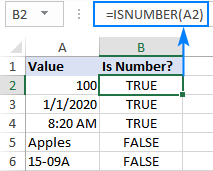### 2 things you should know about ISNUMBER function in Excel

There are a couple of interesting points to note here:

• In internal Excel representation, dates and times are numeric values, so the ISNUMBER formula returns TRUE for them (please see B3 and B4 in the screenshot above).
• For numbers stored as text, the ISNUMBER function returns FALSE (see this example).

## Excel ISNUMBER formula examples

The below examples demonstrate a few common and a couple of non-trivial uses of ISNUMBER in Excel.

### Check if a value is number

When you have a bunch of values in your worksheet and you want to know which ones are numbers, ISNUMBER is the right function to use.

In this example, the first value is in A2, so we use the below formula to check it, and then drag down the formula to as many cells as needed:

`=ISNUMBER(A2)`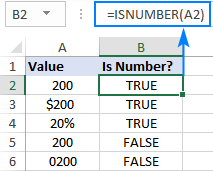Please pay attention that although all the values look like numbers, the ISNUMBER formula has returned FALSE for cells A4 and A5, which means those values are numeric strings, i.e. numbers formatted as text. There may be different reasons for this, for example leading zeros, preceding apostrophe, etc. Whatever the reason, Excel does not recognize such values as numbers. So, if your values do not calculate correctly, the first thing for you to check is whether they are really numbers in terms of Excel, and then convert text to number if needed.

Apart from identifying numbers, the Excel ISNUMBER function can also check if a cell contains specific text as part of the content. For this, use ISNUMBER together with the SEARCH function.

In the generic form, the formula looks as follows:

ISNUMBER(SEARCH(substring, cell))

Where substring is the text that you want to find.

As an example, let's check whether the string in A3 contains a specific color, say red:

`=ISNUMBER(SEARCH("red", A3))`

This formula works nicely for a single cell. But because our sample table (please see below) contains three different colors, writing a separate formula for each one would be the waste of time. Instead, we will refer to the cell containing the color of interest (B2).

`=ISNUMBER(SEARCH(B\$2, \$A3))`

For the formula to correctly copy down and to the right, be sure to lock the following coordinates with the \$ sign:

• In substring reference, lock the row (B\$2) so that the copied formulas always pick the substrings in row 2. The column reference is relative because we want it to adjust for each column, i.e. when the formula is copied to C3, the substring reference will change to C\$2.
• In the source cell reference, lock the column (\$A3) so that all the formulas check the values in column A.

The screenshot below shows the result: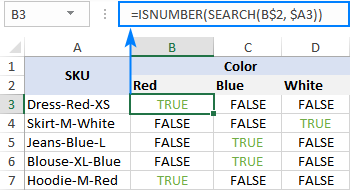#### ISNUMBER FIND - case-sensitive formula

As the SEARCH function is case-insensitive, the above formula does not differentiate uppercase and lowercase characters. If you are looking for a case-sensitive formula, use the FIND function rather than SEARCH.

ISNUMBER(FIND(substring, cell))

For our sample dataset, the formula would take this form:

`=ISNUMBER(FIND(B\$2, \$A3))`

#### How this formula works

The formula's logic is quite obvious and easy to follow:

• The SEARCH / FIND function looks for the substring in the specified cell. If the substring is found, the position of the first character is returned. If the substring is not found, the function produces a #VALUE! error.
• The ISNUMBER function takes it from there and processes numeric positions. So, if the substring is found and its position is returned as a number, ISNUMBER outputs TRUE. If the substring is not found and a #VALUE! error occurs, ISNUMBER outputs FALSE.

### IF ISNUMBER formula

If you aim to get a formula that outputs something other than TRUE or FALSE, use ISNUMBER together with the IF function.

#### Example 1. Cell contains which text

Taking the previous example further, suppose you want to mark the color of each item with "x" like shown in the table below.

To have this done, simply wrap the ISNUMBER SEARCH formula into the IF statement:

`=IF(ISNUMBER(SEARCH(B\$2, \$A3)), "x", "")`

If ISNUMBER returns TRUE, the IF function outputs "x" (or any other value you supply to the value_if_true argument). If ISNUMBER returns FALSE, the IF function outputs an empty string ("").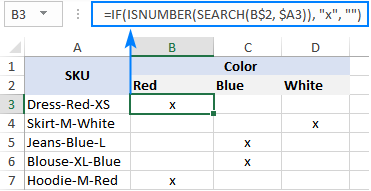#### Example 2. First character in a cell is number or text

Imagine that you are working with a list of alphanumeric strings and you want to know whether a string's first character is a number or letter.

To build such a formula, we you'll need 4 different functions:

• The LEFT function extracts the first character from the start of a string, say in cell A2:

`LEFT(A2, 1)`

• Because LEFT belongs to the category of Text functions, its result is always a text string, even if it only contains numbers. Therefore, before checking the extracted character, we need to try to convert it to a number. For this, use either the VALUE function or double unary operator:

`VALUE(LEFT(A2, 1))` or `(--LEFT(A2, 1))`

• The ISNUMBER function determines if the extracted character is numeric or not:

`ISNUMBER(VALUE(LEFT(A2, 1)))`

• Based on the ISNUMBER result (TRUE or FALSE), the IF function returns "Number" or "Letter", respectively.

Assuming we are testing a string in A2, the complete formula takes this shape:

`=IF(ISNUMBER(VALUE(LEFT(A2, 1))), "Number", "Letter")`

or

`=IF(ISNUMBER(--LEFT(A2, 1)), "Number", "Letter")`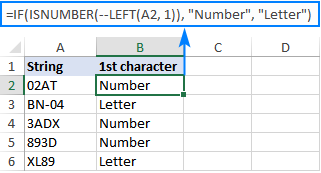The ISNUMBER function also comes in handy for extracting numbers from a string. Here's an example: Get number from any position in a string.

### Check if a value is not number

Though Microsoft Excel has a special function, ISNONTEXT, to determine whether a cell's value is not text, an analogous function for numbers is missing.

An easy solution is to use ISNUMBER in combination with NOT that returns the opposite of a logical value. In other words, when ISNUMBER returns TRUE, NOT converts it to FALSE, and the other way round.

To see it in action, please observe the results of the following formula:

`=NOT(ISNUMBER(A2))`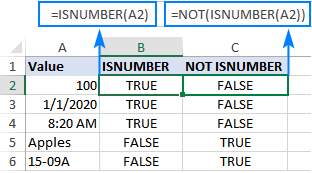Another approach is using the IF and ISNUMBER functions together:

`=IF(ISNUMBER(A2), "", "Not number")`

If A2 is numeric, the formula returns nothing (an empty string). If A2 is not numeric, the formula says it upfront: "Not number".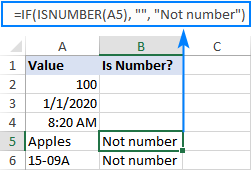If you'd like to perform some calculations with numbers, then put an equation or another formula in the value_if_true argument instead of an empty string. For example, the below formula will multiply numbers by 10 and yield "Not number" for non-numeric values:

`=IF(ISNUMBER(A2), A2*10, "Not number")`

### Check if a range contains any number

In situation when you want to test the whole range for numbers, use the ISNUMBER function in combination with SUMPRODUCT like this:

SUMPRODUCT(--ISNUMBER(range))>0
SUMPRODUCT(ISNUMBER(range)*1)>0

For example, to find out if the range A2:A5 contains any numeric value, the formulas would go as follows:

`=SUMPRODUCT(--ISNUMBER(A2:A5))>0`

`=SUMPRODUCT(ISNUMBER(A2:A5)*1)>0`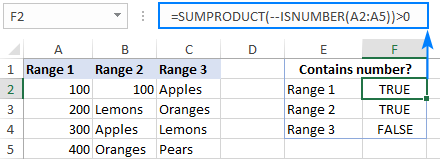If you'd like to output "Yes" and "No" instead of TRUE and FALSE, utilize the IF statement as a "wrapper" for the above formulas. For example:

`=IF(SUMPRODUCT(--ISNUMBER(A2:A5))>0, "Yes", "No")`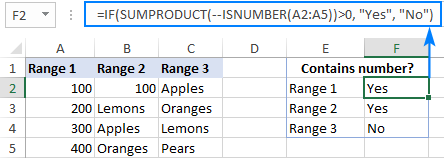#### How this formula works

At the heart of the formula, the ISNUMBER function evaluates each cell of the specified range, say B2:B5, and returns TRUE for numbers, FALSE for anything else. As the range contains 4 cells, the array has 4 elements:

`{TRUE;FALSE;FALSE;FALSE}`

The multiplication operation or the double unary (--) coerces TRUE and FALSE into 1's and 0's, respectively:

`{1;0;0;0}`

The SUMPRODUCT function adds up the elements of the array. If the result is greater than zero, that means there is at least one number the range. So, you use ">0" to get a final result of TRUE or FALSE.

## ISNUMBER in conditional formatting to highlight cells that contain certain text

If you are looking to highlight cells or entire rows that contain specific text, create a conditional formatting rule based on the ISNUMBER SEARCH (case-insensitive) or ISNUMBER FIND (case-sensitive) formula.

For this example, we are going to highlight rows based on the value in column A. More precisely, we will highlight the items that contain the word "red". Here's how:

1. Select all the data rows (A2:C6 in this example) or only the column in which you want to highlight cells.
2. On the Home tab, in the Styles group, click New Rule > Use a formula to determine which cells to format.
3. In the Format values where this formula is true box, enter the below formula (please notice that the column coordinate is locked with the \$ sign):

`=ISNUMBER(SEARCH("red", \$A2))`

4. Click the Format button and choose the format you want.
5. Click OK twice.

If you have little experience with Excel conditional formatting, you can find the detailed steps with screenshots in this tutorial: How to create a formula-based conditional formatting rule.

As the result, all the items of the red color are highlighted: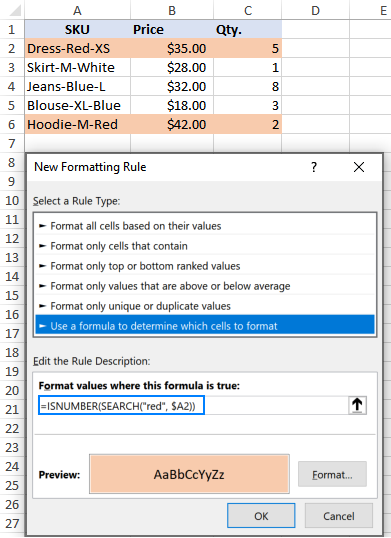Instead of "hardcoding" the color in the conditional formatting rule, you can type it in a predefined cell, say E2, and refer to that cell in your formula (please mind the absolute cell reference \$E\$2). Additionally, you need to check if the input cell is not empty:

`=AND(ISNUMBER(SEARCH(\$E\$2, \$A2)), \$E\$2<>"")`

As the result, you will get a more flexible rule that highlights rows based on your input in E2: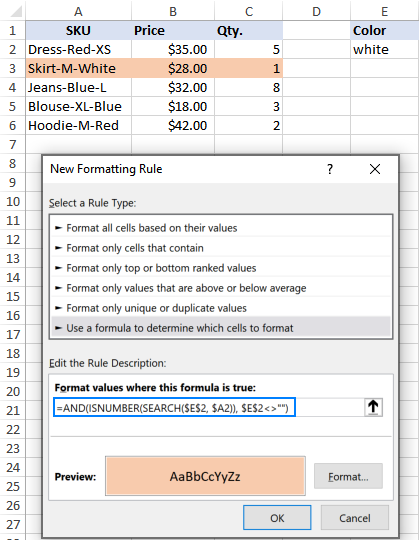That's how to use the ISNUMBER function in Excel. I thank you for reading and hope to see you on our blog next week!